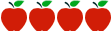### Grades 9-12: Algebra 1 – Descriptive Statistics Module 2

Rating:https://www.engageny.org/resource/algebra-i-module-2

#### Common Core Standards

Summarize, represent, and interpret data on a single count or measurement variable

CCSS.Math.Content.HSS.ID.A.1 Represent data with plots on the real number line (dot plots, histograms, and box plots).

CCSS.Math.Content.HSS.ID.A.2 Use statistics appropriate to the shape of the data distribution to compare center (median, mean) and spread (interquartile range, standard deviation) of two or more different data sets.

CCSS.Math.Content.HSS.ID.A.3 Interpret differences in shape, center, and spread in the context of the data sets, accounting for possible effects of extreme data points (outliers).

CCSS.Math.Content.HSS.ID.A.4 Use the mean and standard deviation of a data set to fit it to a normal distribution and to estimate population percentages. Recognize that there are data sets for which such a procedure is not appropriate. Use calculators, spreadsheets, and tables to estimate areas under the normal curve.

Summarize, represent, and interpret data on two categorical and quantitative variables

CCSS.Math.Content.HSS.ID.B.5 Summarize categorical data for two categories in two-way frequency tables. Interpret relative frequencies in the context of the data (including joint, marginal, and conditional relative frequencies). Recognize possible associations and trends in the data.

CCSS.Math.Content.HSS.ID.B.6 Represent data on two quantitative variables on a scatter plot, and describe how the variables are related.

CCSS.Math.Content.HSS.ID.B.6.A Fit a function to the data; use functions fitted to data to solve problems in the context of the data. Use given functions or choose a function suggested by the context. Emphasize linear, quadratic, and exponential models.

CCSS.Math.Content.HSS.ID.B.6.B Informally assess the fit of a function by plotting and analyzing residuals.

CCSS.Math.Content.HSS.ID.B.6.C Fit a linear function for a scatter plot that suggests a linear association.

Interpret linear models

CCSS.Math.Content.HSS.ID.C.7 Interpret the slope (rate of change) and the intercept (constant term) of a linear model in the context of the data.

CCSS.Math.Content.HSS.ID.C.8 Compute (using technology) and interpret the correlation coefficient of a linear fit.

CCSS.Math.Content.HSS.ID.C.9 Distinguish between correlation and causation.

Standards for Mathematical Practice

MP.1 Make sense of problems and persevere in solving them.

MP.2 Reason abstractly and quantitatively.

MP.3 Construct viable arguments and critique the reasoning of others.

MP.4 Model with mathematics.

MP.5 Use appropriate tools strategically.

MP.6 Attend to precision.

#### Description of Unit

This high school module titled “Algebra I—Descriptive Statistics Module 2” from engageny.org is intended to be completed in 4-5 weeks of 45-minute instructional/assessment sessions. Based on concepts first introduced in grades 6-7-8, students reconnect with and deepen their understanding of statistics and probability. Throughout the module, they develop a set of tools for understanding and interpreting variability in data and begin to make more informed decisions from data.  They work with data distributions of various shapes, centers, and spreads.  Students also build on their experience with bivariate quantitative data from Grade 8.  The culminating task in Lesson 20 has students (individually or in groups) develop a poster that summarizes how a statistical question is answered that involves two numerical variables.  An end-of-module assessment consists of five constructed response questions related to targeted standards. This module sets the stage for more extensive work with sampling and inference in later grades.

#### Cautions

Connecticut teachers should be cautioned that for students to experience success, they must have prior knowledge of the Foundational Standards listed in this module’s documents. Before this module is taught, modifications will be necessary to provide appropriate scaffolding, differentiation, intervention and support for a broad range of learners—including extensions for students with high interest or working above grade level. Lessons would also be enhanced with a mix of instructional approaches. Teachers should be cautioned that some of the activities as described might be difficult to complete in the time prescribed and still achieve the rigor demanded by the Common Core framework. Graphing calculators are required for all students.

#### Rationale for Selection

This module is a good example of mathematical instruction that demonstrates an effective sequence and progression of learning where concepts and skills advance and deepen over time; there is a balance between mathematical procedures and conceptual understanding throughout.  Lessons include clear guidance to support the teaching and learning that engages students in a productive struggle through relevant, thought-provoking questions, problems, and tasks that cultivate student interest and elicit mathematical thinking. The module includes aligned rubrics, answer keys, and/or scoring guidelines that provide sufficient guidance for interpreting student performance.